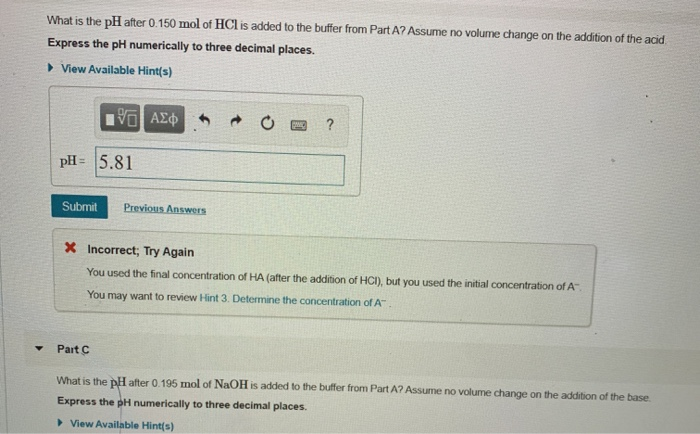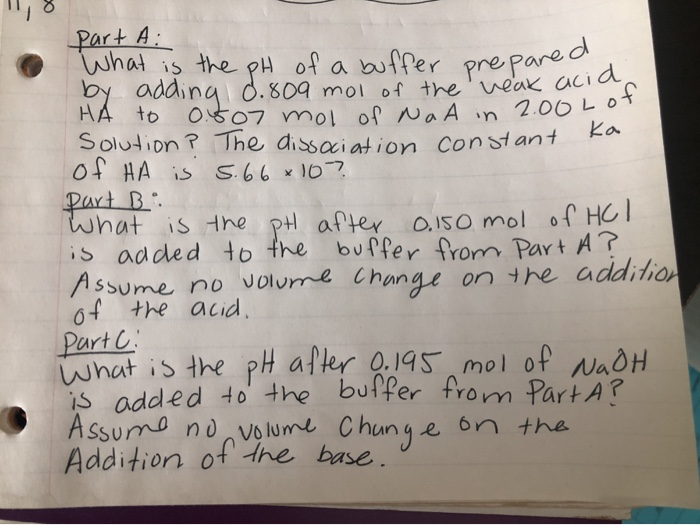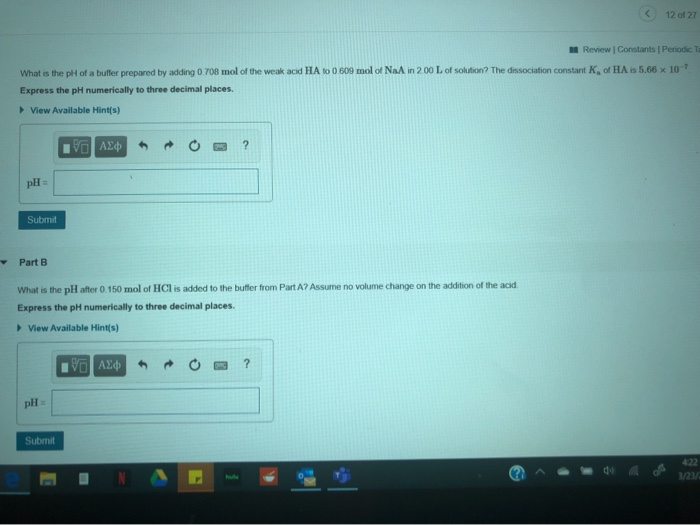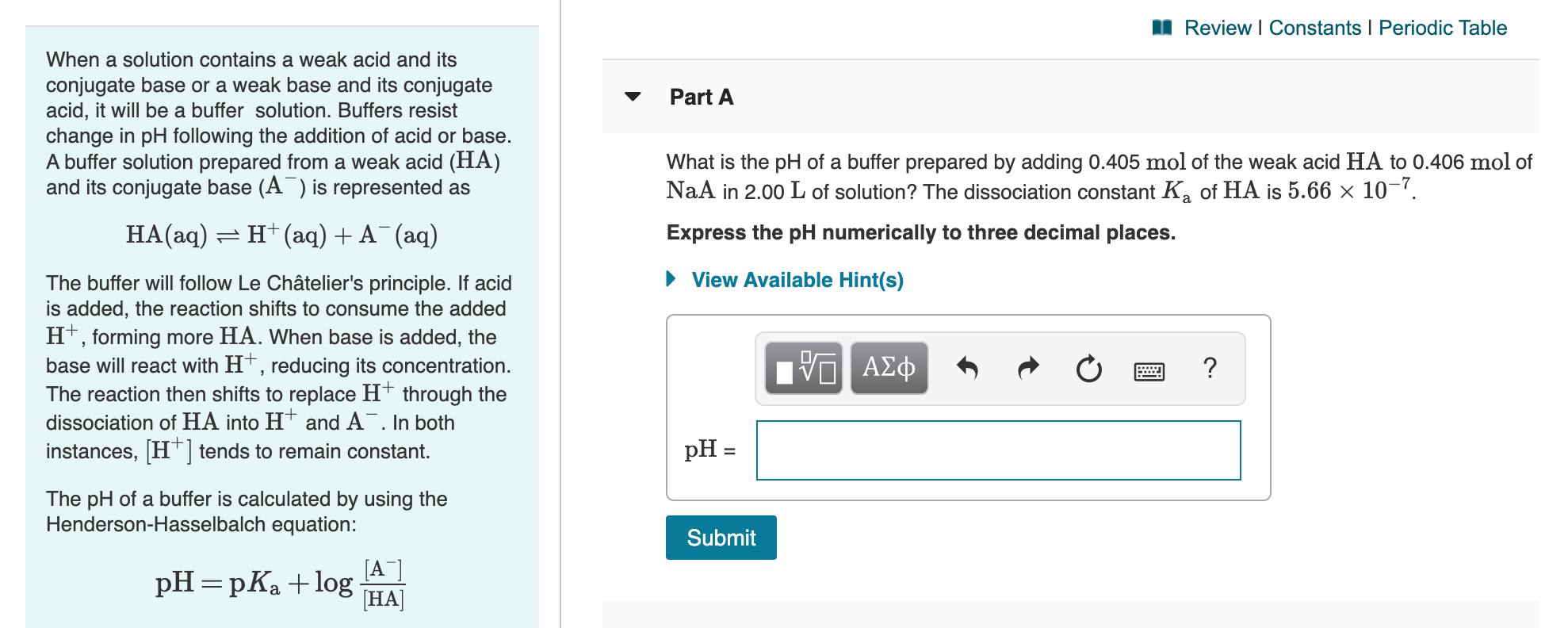# ± pH Changes in Buffers Part A What is the pH of a buffer prepared by...

± pH Changes in Buffers

Part A

What is the pH of a buffer prepared by adding 0.708 mol of the weak acid HA to 0.608 mol of NaA in 2.00 L of solution? The dissociation constant Ka of HA is 5.66×10−7.

Express the pH numerically to three decimal places.

Part B

What is the pH after 0.150 mol of HCl is added to the buffer from Part A? Assume no volume change on the addition of the acid.

Express the pH numerically to three decimal places.

Part C

What is the pH after 0.195 mol of NaOH is added to the buffer from Part A? Assume no volume change on the addition of the base.

Express the pH numerically to three decimal places.

View Available Hint(s)

part A

pH of acidic buffer = pka + log(NaA/HA)

pka =- log(5.66*10^-7) = 6.25

pH = 6.25+log(0.608/0.708)

= 6.184

part B

No of mol Of HCl = 0.15 mol

pH of acidic buffer = pka + log((NaA-HCl)/(HA+HCl))

= 6.25+log((0.608-0.15)/(0.708+0.15))

= 5.977

part C

No of mol Of NaOH = 0.195 mol

pH of acidic buffer = pka + log((NaA+NaOH)/(HA-NaOH))

= 6.25+log((0.608+0.195)/(0.708-0.195))

= 6.444

#### Earn Coin

Coins can be redeemed for fabulous gifts.

Similar Homework Help Questions
• ### Part A What is the pH of a buffer prepared by adding 0.708 mol of the weak acid HA to 0.406 mol of NaA in 2.00 Lof sol...

Part A What is the pH of a buffer prepared by adding 0.708 mol of the weak acid HA to 0.406 mol of NaA in 2.00 Lof solution? The dissociation constant Ka of HA is 5.66×10−7. Express the pH numerically to three decimal places. Part B What is the pH after 0.150 mol of HCl is added to the buffer from Part A? Assume no volume change on the addition of the acid. Express the pH numerically to three decimal...

• ### Part A What is the pH of a buffer prepared by adding 0.506 mol of the weak acid HA to 0.608 mol of NaA in 2.00 L of so...

Part A What is the pH of a buffer prepared by adding 0.506 mol of the weak acid HA to 0.608 mol of NaA in 2.00 L of solution? The dissociation constant Ka of HA is 5.66×10−7. Express the pH numerically to three decimal places. pH = SubmitHintsMy AnswersGive UpReview Part Part B What is the pH after 0.150 mol of HCl is added to the buffer from Part A? Assume no volume change on the addition of the acid....

• ### Part A: What is the pH of a buffer prepared by adding 0.708 mol of the...

Part A: What is the pH of a buffer prepared by adding 0.708 mol of the weak acid HA to 0.406 mol of NaA in 2.00 L of solution? The dissociation constant Ka of HA is 5.66×10−7. Part B:What is the pH after 0.150 mol of HCl is added to the buffer from Part A? Assume no volume change on the addition of the acid. Part C: What is the pH after 0.195 mol of NaOH is added to the...

• ### What is the pH after 0.150 mol of HCl is added to the buffer from Part...What is the pH after 0.150 mol of HCl is added to the buffer from Part A? Assume no volume change on the addition of the acid Express the pH numerically to three decimal places. ► View Available Hint(s) ROO? V AE pH= 5.81 Submit Previous Answers X Incorrect; Try Again You used the final concentration of HA (after the addition of HCI), but you used the initial concentration of A You may want to review Hint 3. Determine the...

• ### Finding pH, using Henderson-Hasselbalch, buffer.

PART A:What is the pH of a buffer prepared by adding 0.809 mol of the weak acid HA to 0.305 mol of NaA in 2.00L of solution? The dissociation constant Ka of HA is 5.66 *10^-7PART B:What is the pH after 0.150 mol of HCl is added to the buffer from Part A? Assume no volume change on the addition of the acid.PART C:What is the pH after 0.195 mol of NaOH is added to the buffer from Part A?...

• ### II, 8 part A: What is the pH of a buffer prepared by adding 0. 809...II, 8 part A: What is the pH of a buffer prepared by adding 0. 809 mol of the veak acid HA to 0.607 mol of Na A in 2.00 LOT Solution? The dissociation constant of HA is 5.66 x 10? Dart B What is the pH after 0.150 mol of HCl is added to the buffer from Part A? Assume no volume change on the addition of the acid. What is the pH after 0.195 mol of NaOH is...

• ### ( 12 0127 Review Constants Periodic T What is the ph of a butler prepared by...( 12 0127 Review Constants Periodic T What is the ph of a butler prepared by adding you mol of the weak acid HA 10 0 609 mol of NaA in 200 L of solution? The dissociation constant of HAI 5.66 x 10 Express the pH numerically to three decimal places View Available Hints) AED + O ? Submit Part B What is the pH after 0. 150 mol of HCl is added to the butter from PartA? Assume no...

• ### What is the pH of a buffer prepared by adding 0.506 mol of the weak acid HA to 0.305 mol of NaA in 2.00  L of solu...

What is the pH of a buffer prepared by adding 0.506 mol of the weak acid HA to 0.305 mol of NaA in 2.00  L of solution? The dissociation constant Ka of HA is 5.66*10^-7. pH = __________ What is the \rm pH after 0.150 mol of \rm HCl is added to the buffer from Part A? Assume no volumechange on the addition of the acid. pH = _____________ What is the \rm pH after 0.195 mol of \rm NaOH...

• ### M Review | Constants | Periodic Table - Part A When a solution contains a weak...M Review | Constants | Periodic Table - Part A When a solution contains a weak acid and its conjugate base or a weak base and its conjugate acid, it will be a buffer solution. Buffers resist change in pH following the addition of acid or base. A buffer solution prepared from a weak acid (HA) and its conjugate base (A) is represented as What is the pH of a buffer prepared by adding 0.405 mol of the weak acid...

• ### change in Ph in buffers part A What is the pH of a buffer prepared by adding 0.607mol of the weak acid HA to 0.608mol...

change in Ph in buffers part A What is the pH of a buffer prepared by adding 0.607mol of the weak acid HA to 0.608mol of NaA in 2.00 L of solution? The dissociation constant Ka of HA is 5.66